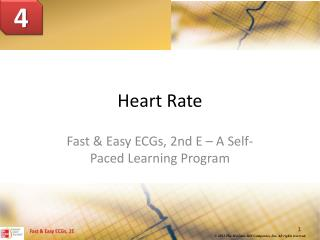DownloadDownload PresentationHeart Rate

Heart Rate

Télécharger la présentationHeart Rate

- - - - - - - - - - - - - - - - - - - - - - - - - - - E N D - - - - - - - - - - - - - - - - - - - - - - - - - - -
Presentation Transcript

1. 4 Heart Rate Fast & Easy ECGs, 2nd E – A Self-Paced Learning Program

2. Dysrhythmias • Irregularities in heart rate or rhythm • Some are of little significance whereas others are life threatening I

3. Determining the Heart Rate • Is one of first steps in analyzing an ECG tracing I

4. Determining the Heart Rate • Begin by quickly checking ECG monitor or tracing to see if rate is slow, normal or fast

5. Calculating Heart Rate • Several methods can be used including: • 6-Second Interval x 10 Method • 300, 150, 100, 75, 60, 50 Method • 1500 Method • Rate Calculator I

6. 6-Second Interval x 10 Method • Quick, easy and does not require tools or devices • Multiply by 10 the number of QRS complexes found in a 6 second portion of the ECG tracing I

7. Practice Makes Perfect • For this tracing, determine the heart rate using the 6-second interval x 10 method I

8. Practice Makes Perfect • For this tracing, determine the heart rate using the 6-second interval x 10 method I

9. Practice Makes Perfect • For this tracing, determine the heart rate using the 6-second interval x 10 method I

10. 300, 150, 100, 75, 60, 50 Method • Quick, fairly accurate, requires no special tools, or calculations • Cannot be used with irregular rhythms • Find an R wave located on a bold line. Then find the next consecutive R wave. Bold line it falls on (or is closest to) represents the heart rate

11. 300, 150, 100, 75, 60, 50 Method • If the 2nd R wave does not fall on a bold line the heart rate can be approximated In this example: the 2nd R waves falls between the 4th and 5th bold line. As such the heart rate is between 60 and 75 BPM

12. 300, 150, 100, 75, 60, 50 Method • If the second R wave falls in between two bold lines the heart rate can be more precisely determined using the identified values for each thin line I

13. Practice Makes Perfect • For this tracing, determine the heart rate using the 300, 150, 100, 75, 60, 50 method I

14. Practice Makes Perfect • For this tracing, determine the heart rate using the 300, 150, 100, 75, 60, 50 method I

15. Practice Makes Perfect • For this tracing, determine the heart rate using the 300, 150, 100, 75, 60, 50 method I

16. 1500 Method • Most accurate and requires no special tools but math calculation must be done to determine heart rate • Cannot be used with irregular rhythms • Count the number of small squares between two consecutive R waves and divide 1500 by that number I

17. Practice Makes Perfect • For this tracing, determine the heart rate using the 1500 method I

18. Practice Makes Perfect • For this tracing, determine the heart rate using the 1500 method I

19. Practice Makes Perfect • For this tracing, determine the heart rate using the 1500 method I

20. Rate Calculators • Easy to use but not always available • Ineffective on irregular rhythms where a consistent baseline is not present • Position the “start mark” on an R wave • Then find the next consecutive R wave – where it lines up is the approximate heart rate

21. Heart Rates • Average adult has a heart rate of 60-100 BPM • Heart rate < 60 BPM called bradycardia • Heart rate > 100 BPM called tachycardia I

22. Summary • One of the 9 steps to analyzing an ECG rhythm is to determining the heart rate • To determine the heart rate first check to see if the rate is slow, normal or fast • Several techniques can be used to determine the heart rate I

23. Summary • The 6-second interval x 10 method multiplies by 10 the number of QRS complexes found in a 6-second portion of the ECG tracing • The 300, 150, 100, 75, 60, 50 method involves locating an R wave on a bold line on the ECG paper, then finding the next consecutive R wave and using the 300, 150, 100, 75, 60, 50 values for subsequent bold lines to determine the rate • To use the 1500 method count the number of small squares between two consecutive R waves and divide 1500 by that number I

24. Summary • A heart rate less than 60 beats per minute is called bradycardia • A heart rate greater than 100 beats per minute is called tachycardia I